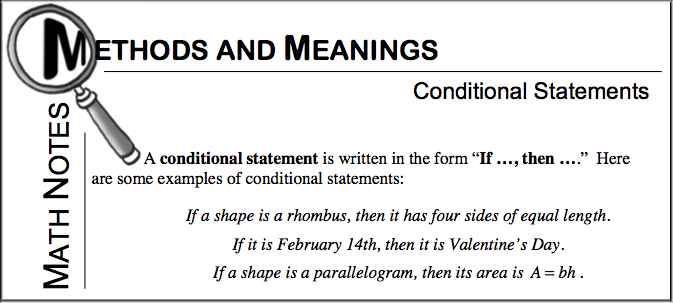### Home > GC > Chapter 2 > Lesson 2.3.1 > Problem2-103

2-103.

Examine the arrow diagram below.

Polygon is a parallelogramarea of the polygon equals base times height.

1. Write this conjecture as a conditional (“If, then”) statement.

2. Write a similar conjecture about triangles, both as a conditional statement and as an arrow diagram.If a polygon is a parallelogram, then its area equals its base times its height.

If a polygon is a triangle, then its area equals one half its base times its height.